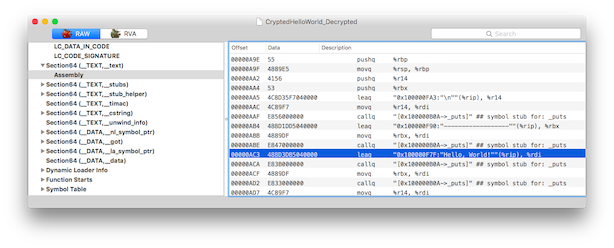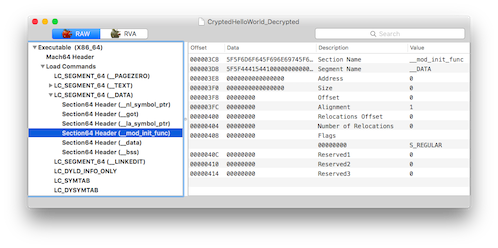In a previous post CryptedHelloWorld: App with encrypted mach-o sections, I created a simple macOS app `CryptedHelloWorld` with its `(__TEXT, __text)` section encrypted. The section is decrypted by a constructor function.

This post explains how to dump the decrypted app. A common way is to attach the app with a debugger (GDB, LLDB) and manually dump the decrypted memory to disk.

However I will use a different solution by using 2 techniques already presented in previous posts: a destructor function and code injection.

The targeted app is the precompiled CryptedHelloWorld command line tool that can be downloaded here.

This command line tool has its `(__TEXT, __text)` section encrypted. Once its main() function is called, we know that the section is decrypted. Thus we can create a destructor function - which is called just before the app is quit - to dump the decrypted memory to disk. This destructor function will be injected into the app using the `DYLD_INSERT_LIBRARIES` environment variable.

The destructor function needs to read the executable from disk, dump the decrypted `(__TEXT, __text)` section and replace the encrypted bytes by the decrypted bytes.

# Code injection

I already described how to inject code using `DYLD_INSERT_LIBRARIES` in this post. We will use the exact same technique by building a dynamic library like this:

``````gcc -o DumpBinary.dylib -dynamiclib DumpBinary.c
``````

and then run it using the `DYLD_INSERT_LIBRARIES` environment variable:

``````DYLD_INSERT_LIBRARIES=./DumpBinary.dylib ./CryptedHelloWorld
``````

# Destructor function

Creating a destructor function has been described in this post. Such a function will be called just before the app quits:

``````void __attribute__((destructor)) DumpBinaryDestructor()
{
// Executed just before the app quits
}
``````

# Finding the targeted app mach-o header

The first problem to solve is to find the mach-o header of the targeted app. This is easily done using the dyld function `_dyld_get_image_header` and searching for the first image of type `MH_EXECUTE`:

``````//
// Find the main executable
//

for(uint32_t imageIndex = 0 ; imageIndex < _dyld_image_count() ; imageIndex++)
{
if (mH->filetype == MH_EXECUTE)
{
const char* imageName = _dyld_get_image_name(imageIndex);
fprintf(stderr, "Found main executable '%s'\n", imageName);

break;
}
}
``````

# Finding the executable path on disk

The dynamic library will need to read the app binary from disk: it needs the executable path. This is done using the dyld function `_NSGetExecutablePath` which copies the path of the main executable into a buffer:

``````//
// Get the real executable path
//
char executablePath[PATH_MAX];

/*
_NSGetExecutablePath() copies the path of the main executable into the
buffer buf.  The bufsize parameter should initially be the size of the
buffer.  This function returns 0 if the path was successfully copied, and
* bufsize is left unchanged.  It returns -1 if the buffer is not large
enough, and * bufsize is set to the size required.  Note that
_NSGetExecutablePath() will return "a path" to the executable not a "real
path" to the executable.  That is, the path may be a symbolic link and
not the real file. With deep directories the total bufsize needed could
be more than MAXPATHLEN.
*/
uint32_t len = sizeof(executablePath);
if (_NSGetExecutablePath(executablePath, &len) != 0)
{
fprintf(stderr, "Buffer is not large enough to copy the executable path\n");
exit(1);
}
``````

We then get the canonical path using `realpath`:

``````//
// Get the canonicalized absolute path
//
char *canonicalPath = realpath(executablePath, NULL);
if (canonicalPath != NULL)
{
strlcpy(executablePath, canonicalPath, sizeof(executablePath));
free(canonicalPath);
}
``````

Reading from disk is done using `fopen`/`fread`:

``````//
// Open the executable file for reading
//
FILE *sourceFile = fopen(executablePath, "r");
if (sourceFile == NULL)
{
fprintf(stderr, "Error: Could not open executable path '%s'\n", executablePath);
exit(1);
}

//
// Read the source file and store it into a buffer
//
fseek(sourceFile, 0, SEEK_END);
long fileLen = ftell(sourceFile);
fseek(sourceFile, 0, SEEK_SET);

uint8_t *fileBuffer = (uint8_t *)calloc(fileLen, 1);
if (fileBuffer == NULL)
{
fprintf(stderr, "Error: Could not allocate buffer\n");
exit(1);
}

if (fread(fileBuffer, 1, fileLen, sourceFile) != fileLen)
{
fprintf(stderr, "Error: Could not read the file '%s'\n", executablePath);
exit(1);
}
``````

# Finding the `(__TEXT, __text)` and `(__DATA, __mod_init_func)` sections

We already have the mach-o header. We need to loop through all segments and sections until we find the interesting sections:

``````//
// Loop through each section
//

for (uint32_t i = 0; i < machHeader->ncmds; i++)
{

{
// Found a 64-bit segment
struct segment_command_64 *segCommand = (struct segment_command_64 *) loadCommand;

// For each section in the 64-bit segment
void *sectionPtr = (void *)(segCommand + 1);
for (uint32_t nsect = 0; nsect < segCommand->nsects; ++nsect)
{
struct section_64 *section = (struct section_64 *)sectionPtr;

fprintf(stderr, "Found the section (%s, %s)\n", section->segname, section->sectname);

if (strncmp(segCommand->segname, SEG_TEXT, 16) == 0)
{
if (strncmp(section->sectname, SECT_TEXT, 16) == 0)
{
// This is the (__TEXT, __text) section.

}
}
else if (strncmp(segCommand->segname, SEG_DATA, 16) == 0)
{
if (strncmp(section->sectname, "__mod_init_func", 16) == 0)
{
// This is the (__DATA, __mod_init_func) section.

}
}

sectionPtr += sizeof(struct section_64);
}
}

}
``````

# Dumping the decrypted `(__TEXT, __text)` section

We just use a simple memcpy to replace in the buffer the encrypted bytes by the decrypted bytes:

``````fprintf(stderr, "\t Save the unencrypted (%s, %s) section to the buffer\n", section->segname, section->sectname);
memcpy(fileBuffer + section->offset, (uint8_t *) machHeader + section->offset, section->size);
``````

# Removing the constructor function

We now have a decrypted binary. However if we launch it, its constructor function will be called and corrupt the `(__TEXT, __text)` section. We need to prevent the constructor function to be executed. There are several solutions and I chose to zero out the `(__DATA, __mod_init_func)` section. I kept the segname and sectname info so that MachOView can nicely display it.

``````fprintf(stderr, "\t Zero out the (%s, %s) section\n", section->segname, section->sectname);

size_t sectionOffset = sectionPtr - (void *)machHeader;

// Size of char sectname + char segname
size_t namesSize = 2 * 16 * sizeof(char);

// Zero out the section_64 but keep the sectname and segname
bzero(fileBuffer + sectionOffset + namesSize, sizeof(struct section_64) - namesSize);
``````

# Writing the decrypted binary to disk

The last step consists of writing the decrypted app to disk. This is done with `fwrite`:

``````//
// Create the output file
//
char destinationPath[PATH_MAX];
strlcpy(destinationPath, executablePath, sizeof(destinationPath));
strlcat(destinationPath, "_Decrypted", sizeof(destinationPath));

FILE *destinationFile = fopen(destinationPath, "w");
if (destinationFile == NULL)
{
fprintf(stderr, "Error: Could create the output file '%s'\n", destinationPath);
exit(1);
}

//
// Save the data into the output file
//
if (fwrite(fileBuffer, 1, fileLen, destinationFile) != fileLen)
{
fprintf(stderr, "Error: Could not write to the output file\n");
exit(1);
}
``````

# Log output

To run the CryptedHelloWorld app and inject our code:

``````DYLD_INSERT_LIBRARIES=./DumpBinary.dylib ./CryptedHelloWorld
``````

Here is the log output when running the CryptedHelloWorld:

``````*** Constructor called to decrypt sections
Found the section (__TEXT, __text)
Decrypting the (__TEXT, __text) section
Found the section (__TEXT, __stubs)
Found the section (__TEXT, __stub_helper)
Found the section (__TEXT, __timac)
Found the section (__TEXT, __cstring)
Found the section (__TEXT, __unwind_info)
Found the section (__DATA, __nl_symbol_ptr)
Found the section (__DATA, __got)
Found the section (__DATA, __la_symbol_ptr)
Found the section (__DATA, __mod_init_func)
Found the section (__DATA, __data)
Found the section (__DATA, __bss)
*** The sections should now be decrypted. main() will be called soon.

------------------
Hello, World!
------------------

*********************************
*** DumpBinaryDestructor CALLED
*********************************
Found main executable '/CryptedHelloWorld/DumpBinary/./CryptedHelloWorld'
Found absolute path: '/CryptedHelloWorld/DumpBinary/CryptedHelloWorld'
Found the section (__TEXT, __text)
Save the unencrypted (__TEXT, __text) section to the buffer
Found the section (__TEXT, __stubs)
Found the section (__TEXT, __stub_helper)
Found the section (__TEXT, __timac)
Found the section (__TEXT, __cstring)
Found the section (__TEXT, __unwind_info)
Found the section (__DATA, __nl_symbol_ptr)
Found the section (__DATA, __got)
Found the section (__DATA, __la_symbol_ptr)
Found the section (__DATA, __mod_init_func)
Zero out the (__DATA, __mod_init_func) section
Found the section (__DATA, __data)
Found the section (__DATA, __bss)
*********************************
*** Decryption completed
*********************************
``````

# Examining the decrypted app

Using MachOView, we see that the `(__TEXT, __text)` section is decrypted:We also see that the `(__DATA, __mod_init_func)` has been zeroed out:# Limitations of the dynamic library

• it only supports 64-bit intel Mach-O files. Adding 32-bit, ARM or fat Mach-O support is fairly simple and left to the reader.
• it only dumps the `(__TEXT, __text)` section.
• it zeroes out the `(__DATA, __mod_init_func)` section which would cause problems if there are multiple constructors.